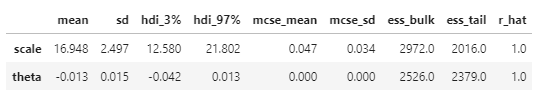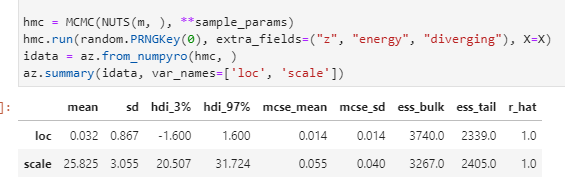# Scan + Condition + TransformReparam

Following the sgt example I set my observed within the `scan` using `handlers.condition`, this works well for a standard distribution but fails for a transformed distribution. Is it possible with NUTS to set the sample for an observed, transformed distribution within a scan?

``````import numpy as np
import numpyro
import numpyro.distributions as dist
from numpyro.contrib.control_flow import scan
from numpyro.infer import NUTS, MCMC, init_to_median, Predictive
from numpyro.distributions.transforms import AffineTransform
from numpyro.infer.reparam import TransformReparam
from jax import random
import arviz as az
import jax.numpy as jnp
import jax
import pandas as pd
numpyro.set_host_device_count(4)
np.set_printoptions(suppress=True)

sample_params = {
'num_chains':4,
'num_warmup':1000,
'num_samples':1000,
'progress_bar':True,
}

#generate AR1 process
N, K = 200, 5
scale = 2.
z = np.random.RandomState(8).normal(scale=scale, size=(N, K))
theta = -0.2
y = np.zeros_like(z)
y[0, :] = z[0, :]
y[1:, :] = z[:-1, :]*theta + z[1:, :]
y = jnp.array(y, dtype=jnp.float32)

def model1(N, K, N_future, y):
scale = numpyro.sample('scale', dist.Exponential(1))
theta = numpyro.sample('theta', dist.Normal(0, 1))

def transition(y_prev, t):
loc = y_prev*theta
y_ = numpyro.sample('y', dist.Normal(loc=loc, scale=scale), )
return y_, y_

init_0 = y
with numpyro.handlers.condition(data={'y': y}):
_, ys = scan(transition, init_0, jnp.arange(N + N_future), )
if N_future > 0:
numpyro.deterministic("y_forecast", ys[-N_future:])

hmc = MCMC(NUTS(model1, target_accept_prob=0.9, init_strategy=init_to_median), **sample_params)
hmc.run(random.PRNGKey(0), extra_fields=("z", "energy", "diverging"), N=N, K=K, y=y, N_future=0)
idata = az.from_numpyro(hmc, )
az.summary(idata)

def model2(N, K, N_future, y):
scale = numpyro.sample('scale', dist.Exponential(1))
theta = numpyro.sample('theta', dist.Normal(0, 1))

def transition(y_prev, t):
loc = y_prev*theta
y_ = numpyro.sample('y',
dist.TransformedDistribution(
dist.Normal(loc=loc, scale=1.),
AffineTransform(0, scale)
)
)
return y_, y_

init_0 = y
with numpyro.handlers.condition(data={'y': y}):
_, ys = scan(transition, init_0, jnp.arange(N + N_future), )
if N_future > 0:
numpyro.deterministic("y_forecast", ys[-N_future:])

hmc = MCMC(NUTS(model2, target_accept_prob=0.9, init_strategy=init_to_median), **sample_params)
hmc.run(random.PRNGKey(0), extra_fields=("z", "energy", "diverging"), N=N, K=K, y=y, N_future=0)
idata = az.from_numpyro(hmc, )
az.summary(idata)

def model3(N, K, N_future, y):
scale = numpyro.sample('scale', dist.Exponential(1))
theta = numpyro.sample('theta', dist.Normal(0, 1))

def transition(y_prev, t):
loc = y_prev*theta
with numpyro.handlers.reparam(config={"y": TransformReparam()}):
y_ = numpyro.sample('y',
dist.TransformedDistribution(
dist.Normal(loc=loc, scale=1.),
AffineTransform(0, scale)
)
)
return y_, y_

init_0 = y
with numpyro.handlers.condition(data={'y': y}):
_, ys = scan(transition, init_0, jnp.arange(N + N_future), )
if N_future > 0:
numpyro.deterministic("y_forecast", ys[-N_future:])

hmc = MCMC(NUTS(model3, target_accept_prob=0.9, init_strategy=init_to_median), **sample_params)
hmc.run(random.PRNGKey(0), extra_fields=("z", "energy", "diverging"), N=N, K=K, y=y, N_future=0)
idata = az.from_numpyro(hmc, )
az.summary(idata)
``````
• model1() runs fine and and recovers the true parameters in my minimal working example.
• model2() fails with error:
`NotImplementedError: Flatenning TransformedDistribution is only supported for some specific cases. Consider using `TransformReparam` to convert this distribution to the base_dist, which is supported in most situtations. In addition, please reach out to us with your usage cases.`
• model3() runs without error but the condition handler doesn’t work to set the observed for `y`

Here I am using AffineTransform which I could easily do without, as in model1(), but it’s just to display the error.

We currently do not have sort of “jit” rule for bijector transform, so we can’t use transform distribution in scan without reparam handler. Please make a feature request for it if you think it is important to have. For model3, it is a pending issue. As a temporary solution, you can condition on `y_base` using `data={'y_base': AffineTransform(0, scale).inv(y)}`.

Thanks for the quick reply, however something isn’t quite working

It runs without error and converges but it doesn’t recover the good values

``````def model3(N, K, N_future, y):
scale = numpyro.sample('scale', dist.Exponential(1))
theta = numpyro.sample('theta', dist.Normal(0, 1))

def transition(y_prev, t):
loc = y_prev*theta
with numpyro.handlers.reparam(config={"y": TransformReparam()}):
y_ = numpyro.sample('y',
dist.TransformedDistribution(
dist.Normal(loc=loc, scale=1.),
AffineTransform(0, scale)
)
)
return y_, y_

init_0 = y
with numpyro.handlers.condition(data={'y_base': AffineTransform(0, scale).inv(y)}):
_, ys = scan(transition, init_0, jnp.arange(N + N_future), )
if N_future > 0:
numpyro.deterministic("y_forecast", ys[-N_future:])

hmc = MCMC(NUTS(model3, target_accept_prob=0.9, init_strategy=init_to_median), **sample_params)
hmc.run(random.PRNGKey(0), extra_fields=("z", "energy", "diverging"), N=N, K=K, y=y, N_future=0)
idata = az.from_numpyro(hmc, )
az.summary(idata, var_names=['scale', 'theta'])
``````

the results are:Taking a simpler example to see if conditioning on the inverse transform works and it seems to not.

``````X = np.random.RandomState(8).normal(loc=0, scale=8, size=200)
def m(X):
loc = numpyro.sample('loc', dist.Normal(0, 1))
scale = numpyro.sample('scale', dist.Exponential(1))
with numpyro.handlers.condition(data={'X': AffineTransform(loc, scale).inv(X)}):
obs = numpyro.sample('X', dist.Normal(0, 1), )

hmc = MCMC(NUTS(m, ), **sample_params)
hmc.run(random.PRNGKey(0), extra_fields=("z", "energy", "diverging"), X=X)
idata = az.from_numpyro(hmc, )
az.summary(idata, var_names=['loc', 'scale'])
``````

It fails to recover the true scale of 8, though it does converge and also move away from the priorOops, sorry, I guess we need an additional jacobian term?

``````    X_base = AffineTransform(loc, scale).inv(X)
with numpyro.handlers.condition(data={'X': X_base}):
obs = numpyro.sample('X', dist.Normal(0, 1), )
sign = 1  # or -1, I'm not sure for now
numpyro.factor('logdet', sign * AffineTransform(loc, scale).log_det_abs_jacobian(X_base, X))
``````
1 Like

You are a maestro, thanks very much. Sign should be -1.

Let me try applying on my real model now.